# Statistical Analysis

Publish Date: Oct 01, 2015 | 8 Ratings | 3.38 out of 5 | Print | Submit your review

### Statistical Analysis

NI LabVIEW software includes an extensive probability and statistics library. Probability is the chance that an event will or will not occur. Statistics, the study of probability, provides information that summarizes data and presents it in an easily interpreted form. You can use the Probability and Statistics VIs in LabVIEW to perform probability, descriptive statistics, analysis of variance, and interpolation functions.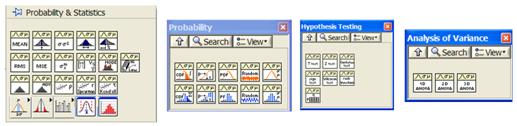Figure 1. Probability and Statistics Library in LabVIEW

The following example uses a data set with 100 values loaded into a numerical array (double data type). Different statistical calculations are required for this data set, using 20 intervals:

• Histogram
• Mean
• Median
• Kurtosis
• Skewness
• Mode
• Variance
• Standard deviation

Note: You can use the File I/O VIs to read/write from/to data files. These files can be of CSV, ASCII, or similar type; binary (LV); or created with Microsoft Excel or another tool. Also, you can directly access databases with LabVIEW either using specific connectivity tools (Active X, .NET, or similar), function libraries compiled as DLLs, or SQL commands. You can also acquire and analyze data in real time using one or more National Instruments data acquisition devices.

First, you can easily calculate the histogram using the corresponding VI: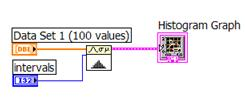Figure 2. Creating a Histogram in LabVIEW

The following histogram is obtained: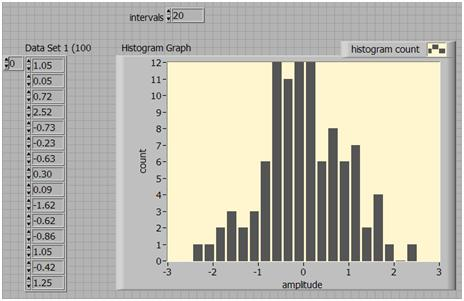Figure 3. A Histogram in LabVIEW

As shown in Figure 3, the data set is listed as an array (partial view); however, you can modify the code to display the data set graphically and as a distribution with 20 intervals. The following code does exactly that: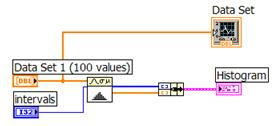Figure 4. Modifying the Code to Display the Data Set Graphically as a Distribution with 20 Intervals

Use a waveform graph and an XY graph to display the data set (left) and the histogram (right).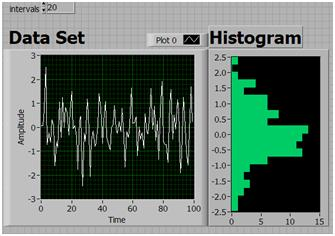Figure 5. Displaying a Data Set and Histogram with a Waveform Graph and an XY Graph

You can easily calculate the standard deviation, mean, and variance using the same VI with the following code (you can define “sample” or “population” for the weighting; “sample” is the default selection):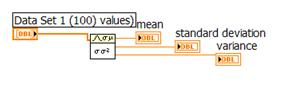Figure 6. Code to Calculate Standard Deviation, Mean, and Variance

The calculated values are as follows: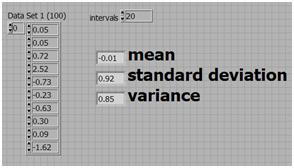Figure 7. Graphic Depiction of Code in Figure 6

There are no specific VIs for calculating the Kurtosis and skewness, but you can easily calculate both using the following equation for the moment about the mean: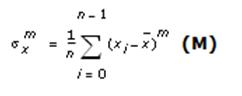where, n is the number of elements in X and x-bar is the mean of X.

The Kurtosis is defined as a measure of peakedness and corresponds to the fourth-order (4th) moment. The skewness is defined as a measure of symmetry and corresponds to the third-order (3rd) moment.

So, to calculate the skewness, you can use the following LabVIEW code: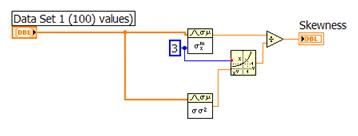Figure 8. Code to Calculate Skewness in LabVIEW

The skewness of the data set is indicated in Figure 9.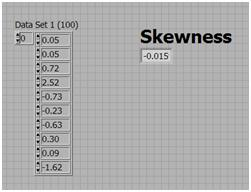Figure 9. Data Set Skewness

In a similar way, by just changing the moment to the fourth order, you calculate the Kurtosis of the data set with the following simple LabVIEW code: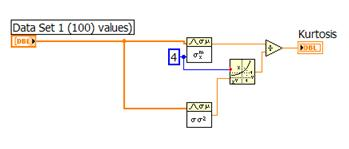Figure 10. Code to Calculate Kurtosis in LabVIEW

The Kurtosis analysis results are shown in Figure 11.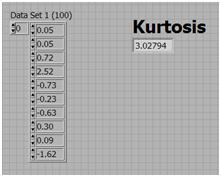Figure 11. Kurtosis Analysis Results

You can calculate the median and mode (unimodal, for 20 intervals) of the data set with the following code: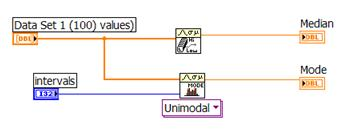Figure 12. Code to Calculate Median and Mode in LabVIEW

The calculated values for mode and median are shown in Figure 13.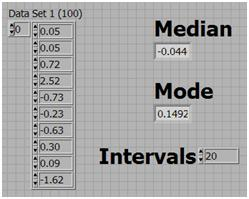Figure 13. Mean and Mode

Additionally, the following combinatorial statistics are required:

• Covariance
• Correlation
• MSE (mean square error)

For this analysis, you need a second data set. Using one single program with a “Case” selector, you can implement the covariance, correlation, and MSE in one single program: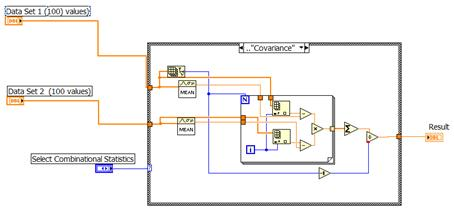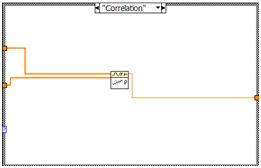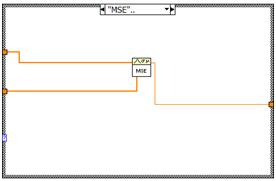Figure 14. Implementing the Covariance, Correlation, and MSE in One Program

The covariance value for the data sets is shown in Figure 15.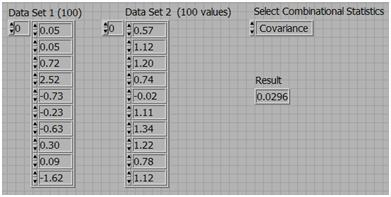Figure 15. Covariance Value of Data Sets

Other functions available in the library include analysis of variance or ANOVA (1D, 2D, and 3D), different probabilistic distributions, and hypothesis testing. Connectivity with statistical analysis packages such as Minitab is also possible.

In addition to all these statistical functions included in LabVIEW, the Statistical Process Control (SPC) Toolkit can add more functionality, including Pareto analysis, control charts (X-bar and R-var), process capability analysis, additional histogram options, and so on. These and other topics are covered in the second part of this tutorial series in "Statistical Analysis with LabVIEW."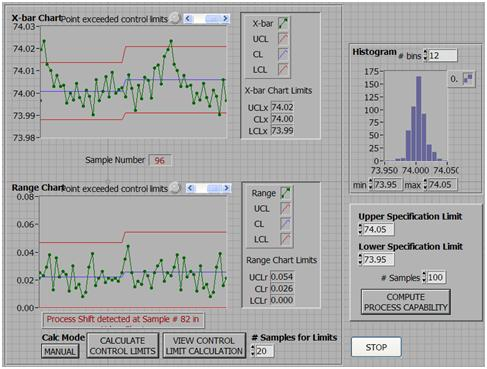Figure 16. An Example of the Statistical Analysis Functions in LabVIEW

You can use other external tools such as NAG (DLLs) with LabVIEW via the Call Library Function VI for additional statistical analysis options. You can incorporate Microsoft Excel, GAUSS, SPSS, Minitab, and other tools with extensive statistical analysis capabilities into LabVIEW through one or more of the connectivity mechanisms mentioned above.

Scientific Computing with Graphical System Design

Introduction to Curve Fitting

Scripting Languages and NI LabVIEW

### Ratings

Rate this document